## Sunday, February 12, 2006

### Euler's Criterion

In today's blog I continue the proof for the Principle of Quadratic Reciprocity. If you are not familiar with the concepts of quadratic reciprocity, quadratic residues, or the Legendre Symbol start here.

If you would like to start this proof at the beginning, start here.

If you are not familiar with the Legendre Symbol, you should review here.

Today's proof is taken from Gareth A. Jones and J. Mary Jones' Elementary Number Theory.

Definition 1: Qn: the set of quadratic residues

That is, these are the integers a mod n where a ≡ s2 (mod n) and s ∈ Un

NOTE: See here for a review of Un

Examples:

Q7 = { 1, 2, 4 }

12 ≡ 1 (mod 7) [ 1 ∈ U7 since 1 ≡ 1 (mod 7) ]
22 ≡ 4 (mod 7) [ 4 ∈ U7 since (4)(2) ≡ 1 (mod 7)]
42 ≡ 2 (mod 7) [ 2 ∈ U7 since (2)(4) ≡ 1 (mod 7)]

Q8 = { 1 }

12 ≡ 1 (mod 8)

Lemma 1: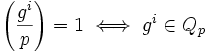Proof:

This is true by the the definition of the Legendre Symbol (see here)

QED

Lemma 2: if p is an odd prime and g is a primitive root for Up, then gi ∈ Qp if and only if i is even.

Proof:

(1) if i is even, then gi/2 ∈ Up [See here for definition of primitive root]

(2) But gi/2 ∈ Up, then gi ≡ (gi/2)2 (mod p) and therefore gi ∈ Qp [See above for definition of Qp]

(3) if gi ∈ Qp, then there exists j such that gi ≡ (gj)2 (mod p) [Based on definition of Qp]

(4) so, i ≡ 2j (mod φ(p)) since:

(a) φ(p) is the order of g. [See detail here]

(b) There exists q,r such that [from the Division Algorithm, see here]:
i = q(φ(p)) + r
0 ≤ r which is less than φ(p)

(c) And, i ≡ r (mod φ(p))

(d) So, gi ≡ gq(φ(p))+r ≡ (gφ(p))q*gr ≡ (1)q*gr ≡ gr (mod p)

(e) There exists, q', r' such that:
2j = q'(φ(p)) + r'
0 ≤ r' which is less than φ(p)

2j ≡ r' (mod φ(p))

(f) Now it follows that r = r' since:
g2j ≡ (1)q'*gr' ≡ gr' ≡ gr (mod p)

(g) Putting this all together, gives us:
2j ≡ i ≡ r (mod p)

(5) Now φ(p) is even. [since φ(p) = p-1 and p is an odd prime -- see here for details.]

(6) So i is even since:

(a) There exists a such that φ(p) = 2a [Since φ(p) is even]

(b) There exists b such that i - 2j = (2a)b [By definition of modular arithmetic, see here]

(c) So, 2 must divide i.

QED

Lemma 3: If p is an odd prime and g is a primitive root mod p, then:Proof:

(1) Both values are either equal to 1 or -1. [See here for definition of Legendre Symbol]

(2) So, I will prove that: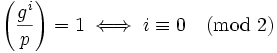In other words, it is 1 only if i is even.

(3) In order for it to be 1, gi must be an element of Qp. [See Lemma 1 above]

(4) From Lemma 2, we know that gi ∈ Qp if and only if i is even.

QED

Theorem 1: Euler's Criterion: if p is an odd prime and a is any integer then: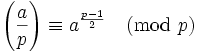Proof:

(1) We can assume that p does not divide a since if it did: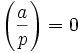and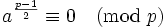which proves the conclusion.

(2) Now, if p doesn't divide a, then gcd(p,a)=1 since p is a prime.

(3) Also, there exists a' such that a ≡ a' (mod p) and a' is a unit mod p since:

(a) gcd(p,a)=1 → there exists u,v such that au + pv = 1 (By Bezout's Identity, see here for proof)

(b) So, au - 1 = pv → au ≡ 1 (mod p)

(c) But then a is a unit mod p (see here for definition of a unit mod p)

(4) The set of units mod p is cyclic (see here for proof)

(5) Then, there exists an element g such that g is the primitive root for the set of units mod p. (see here for definition of cyclic)

(6) So, there exists i such that a ≡ gi (mod p) (see here for definition of primitive root)

(7) Let h ≡ g(p-1)/2 (mod p)

(8) h2 ≡ gp-1 (mod p) [See here for a review of exponents if needed]

(9) φ(p) = p-1 [See here for details.]

(10) gp-1 ≡ gφ(p) ≡ 1 (mod p) [See Euler's Theorem]

(11) So p divides gp-1-1 → p divides h2 - 1 → p divides (h-1)(h+1)

(12) So, h ≡ 1 or h ≡ -1 (mod p)

(13) But h cannot be ≡ 1 (mod p) since (p-1)/2 is less than p-1 and p-1 is the order [See here for the definition of order]

(14) So h ≡ -1 (mod p)

(15) From (#6), we have: a(p-1)/2 ≡ (gi)(p-1)/2 ≡ (g(p-1)/2)i ≡ hi ≡ (-1)i (mod p)

(16) Now from Lemma 3 above:(17) Since a ≡ gi (mod p), we also have:(18) Finally, since a(p-1)/2 ≡ (-1)i (mod p) from#15, we have: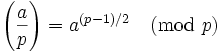QED